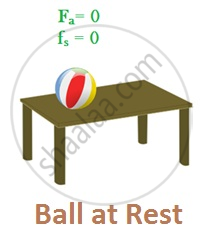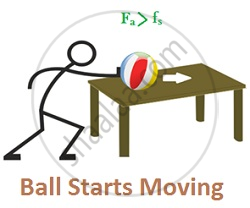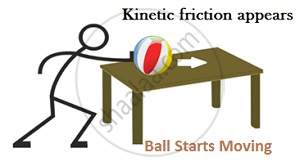# Static and Kinetic Friction

## Static friction

• Static friction is the force of friction between two surfaces so long as there is no relative motion between them. It is always equal to the applied force.

• Force that resists initiation of motion of one body over another with which it is in contact

• Opposes Impending motion

• Denoted by fs

Let the ball be at rest initially
Applied force, Fa = 0; Static friction, fs = 0Later, applied force, Fa = F, then fs also increases but only up to a certain limit. As soon as Fa becomes greater than fs, the ball starts to move.Fs acts when a body is at rest. Hence called Static friction.

• Limiting value of fs depends on Normal reaction, and is independent of the area of contact

fs max ∝ N
fs max = constant × N
fs max = μs N
where μs is the coefficient of static friction
This coefficient depends on the nature of surfaces in contact.

• According to the Law of Static friction, Static friction is always less than or equal to the limiting value of fs

fs =< fs max
fs max = μs N
fs =< μs N

## Kinetic friction

• Kinetic friction is the force of friction which comes into play between two surfaces when there is some relative motion between them.

• Force that resists motion of one body over another with which it is in contact.

• Denoted by fk

• As motion starts, fs vanishes and fk appearsKinetic friction is Independent of the area of contact and velocity of the body

• It varies with Normal reaction, N

fk ∝ N
fk = constant × N
fk = μk N; μk is the coefficient of kinetic friction

Three scenarios can arise in a body’s motion
1. When applied force > fk
Fa > fk
(Fa – fk) = ma
a = (Fa – fk)/m

2. When applied force = f
Fa = fk
Therefore, a = 0 i.e. the body moves with uniform velocity

3. When applied force = 0
Fa = 0
A = - fk/m
No motion occurs, the body stops

Relation between Coefficient of Static & Kinetic friction
fk = μk N
fs =< μs N
fs > fk (to keep the body moving)
μs N > μk N
Or, μk < μs
Coefficient of kinetic friction is smaller than the coefficient of static friction.

If you would like to contribute notes or other learning material, please submit them using the button below.